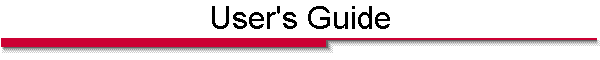Home | Contact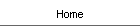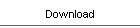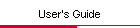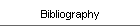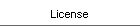The SMTP user's guide is also included in PDF format in the downloaded zip archive.

# Chapter 1 Getting Started

The Source-Model Technique Package (SMTP) is a collection of MATLAB scripts and functions for determining the modes of dielectric waveguides. The solution method is based on the Source-Model Technique (SMT) as described in Refs. [1,2,3,4]. Many of the functions of the package can be accessed via a Graphical User Interface (GUI), by typing smtgui at the MATLAB prompt. All functions can be accessed through MATLAB code which can be used to integrate the SMT solver with other MATLAB programs.

## 1.1  What's new in SMTP v2.0

The main things that are new in version 2.0 are the following: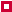The mode search algorithm has been replaced with a new algorithm that is more robust and can deal with degenerate and nearly-degenerate modes. It can also search the complex plane from the smtgui.The eigenvalue determination algorithm has been improved in order to avoid inaccuracies that could lead to spurious minima. Also, an 'indirect' Generalized Singular Value Decomposition (GSVD) method which is slower but even more robust has been made available.Circles and ellipses are not approximated by splines; their exact representation is used. This requires that older (i.e., SMTP v1.0) geometry descriptors be updated with the function updateGd.A few examples and a new reference  have been added. Reference  should become the main reference for the SMTP. If you use this package, we kindly ask that you cite this reference.James Trevelyan's package has been integrated to allow geometries to be exported to the AutoCAD DXF format (readable by other electromagnetic analysis software such as COMSOL).Online help has been added to the smtgui.A new function, out2curves, that sorts the smtgui output into a family of dispersion curves has been added.A few bugs of the GUI have been fixed.

## 1.2  Installation Instructions

To install the package, unzip its contents to a new folder and add the folder to the MATLAB path with the setpath command.

The SMTP uses a library called 'pointer' by Nikolai Yu. Zolotykh. This library includes files in C which must be compiled before being run. They are compiled by the MATLAB mex command. The SMTP includes a compiled version of the library files for Windows. In other operating systems, however, this library must be compiled before using the SMTP. See instructions for compiling the library in the file: @pointer\contents.m.

### 1.2.1  System Requirements

The SMTP runs on MATLAB 7.x. Owing to differences in the format of MAT and FIG files, you will not be able to run it on older versions of MATLAB . It requires the 'image processing' and 'spline' toolboxes.

## 1.3  How to avoid reading the rest

Some people like to experiment with a program as a way of acquainting themselves with it, instead of reading a manual. The smtgui is quite user-friendly and you are encouraged to try it out. Also, it has a 'Command-line window' which indicates how the calculations could be made from the MATLAB command-line. All the settings of the program, including the geometry used and the results obtained, may be saved in a session file. A few session files are supplied with the package, to allow the user to quickly set all parameters to suitable values for sample problems. The following session files are supplied with the package:

 step_index.se a few modes of a round step-index fiber analyzed in Ref.  cookie.se the fundamental mode of a cookie-shaped dielectric fiber made of silica. mcphedran.se the fundamental mode of a holey fiber model analyzed in Ref.  realistic.se the fundamental mode of a realistic holey fiber analyzed in Ref.  touching.se a few modes of two touching round step-index fibers analyzed in Ref. . nanowire.se a surface plasmon-polariton on a round silver nanowire analyzed in Ref. . sensor.se the fundamental mode of a holey fiber proposed as a sensor in Ref.. almost_circular.se all the modes of an almost-circular (elliptical) step-index fiber analyzed in Ref. .

After typing smtgui at the prompt, open these files by pressing the 'Open Session/Geometry' button on the toolbar. Set the file type to 'Session File', and select the file from the list. To find the modes press the 'Find Modes' button on the toolbar. Then plot the fields with the 'Plot Fields' button.

To create a new geometry, press the 'Geometry from Image' button, and follow the instructions in the dialog. The geometry is created in this way from a bitmap image. Alternatively, geometries may be constructed by MATLAB code. The following scripts are included in the package, and can be used to learn how to create geometries with code:

 make_step_index.m create the geometry used in step_index.se make_cookie.m create the geometry used in cookie.se make_mcphedran.m create the geometry used in mcphedran.se make_realistic.m create the geometry used in realistic.se (requires the file realistic_curves.mat). make_touching.m create the geometry used in touching.se make_nanowire.m create the geometry used in nanowire.se make_sensor.m create the geometry used in sensor.se (requires the file sensor_curves.mat). make_almost_circular.m create the geometry used in almost_circular.se

There are a number of functions that can be used to run the solver using MATLAB code. To learn how to use these, you may look at the driver scripts, which are scripts that call the functions with the appropriate parameters. The driver scripts are:

 smt_solve_drv_proper.m scan complex neff plane for proper modes. smt_solve_drv_improper.m scan complex neff plane for improper modes. dispersion.m calculate dispersion curves. calc_field_drv.m plot modal fields. calc_att_drv.m calculate confinement losses with a perturbational method.

# Chapter 2 Using the Graphical User Interface

## 2.1  Creating a geometry from an image

In the SMTP, geometries are defined by boundary curves that are represented by cubic splines. To create these splines from a bitmap image, press the 'Geometry From Image' button, or select it from the 'File' menu. A dialog will appear asking for an image file. The file types supported are those supported by MATLAB . Select an image of the geometry. The image will be thresholded, and the boundaries between dark and light areas will be used to generate the boundaries of the geometry. The program uses a fraction of the points on the boundaries as sampling points through which the spline must pass. This fraction may be varied with the slider control. Generally, you will want to use enough points so that the spline resembles sufficiently the actual geometry. On the other hand, if you use too many points the boundary will be artificially complicated, due to noise and the finite sampling resolution of the bitmap image. Press the 'Find curves' button and move the slider until a good representation of the boundaries is obtained. Then set the scaling factors in X and Y and press the 'Next' button.

## 2.2  Setting the material parameters

In the next dialog the material parameters of the geometry are set. This dialog can be brought up later to change these parameters by pressing the 'Set Material Parameters' in the toolbar. The program begins by analyzing the geometry to find all the sub-domains. These regions must be filled with a homogenous material having the permeability of free-space. The complex permittivities can be set to the desired values by selecting the regions with the mouse, and setting the values in the edit-box. Note that the assumed time dependence is exp(j wt). When using the list to select the regions, multiple region selection is possible by holding down the SHIFT or CTRL keys while selecting the regions.

To take into account material dispersion, i.e. a frequency dependent permittivity, the name of a MATLAB function may be written in the permittivity edit-box. This function should accept one input argument, the free-space wavelength in meters, and should return the complex permittivity at that wavelength. A small number of such functions are provided:

 gold.m For noble metals: copper.m Interpolation of experimental values silver.m given by Johnson and Christy  aluminum.m A simple Drude model sellmeier.m Sellmeier equation for fused silica.

These functions must be in the MATLAB path. When all the permittivities are set, press the 'Next' button.

## 2.3  Setting the source and testing points parameters

In this dialog, the number and locations of sources and testing points are set. The dialog can be brought up later by pressing the 'Set Source Parameters' button from the toolbar. The program will automatically set the number of sources and testing points to default values, that should work in the most common cases. To verify that a solution is correct you will want to increase the number of sources and testing points and see that the solution converges and that the error in continuity conditions decreases.

### 2.3.1  Guidelines for source and testing-point location

Deciding on the number of sources, testing points, and their location is an important issue. As a rule, the number of sources should be enough to approximate the fields with high enough fidelity. This means that the anticipated spatial variation of the fields should be used as a guideline for the number of sources necessary. An important length in this context is the transversal wavelength, which is given by,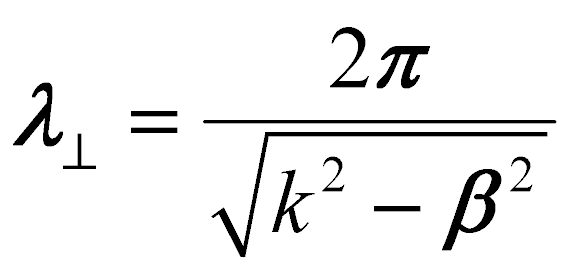(2.1)

where k is the wave-number in the material and b the longitudinal propagation constant. Another important length is the radius of curvature of the boundary. The smaller one of these two lengths can be used to estimate the spatial variation of the fields; a few sources per radius of curvature, or transversal wavelength are usually enough.

The distance of the sources from the boundaries is measured in units of the minimal radius of curvature of each curve. In this way, the fields near curves which have finer details are approximated by sources which are placed more closely to the curve. As a rule, when the sources approach the boundary their number must be increased, because otherwise it is difficult to approximate a field which varies smoothly on the boundary. Note that curves which have sharp corners lead to sources placed very near them. Corners should in general be dealt with separately. At the moment, this is a limitation of the SMTP. It cannot handle sharp corners well.

The number of testing points should be greater, by a factor of about 1.3, than the number of sources.

When the sources and testing points are distributed satisfactorily, press the 'Next' button. You will be asked to save the geometry in a Geometry Description File (GD file), in which all the information entered in the previous dialogs is stored. The filename should have a 'gd' extension.

## 2.4  Opening and Saving a geometry or session

For opening, press the 'Open Geometry/Session' button. You may choose to either open a GD file or a session file, by changing the file type. Session files store all the settings and results of the program. Note that a session filename should have an 'se' extension. Press the 'Save Session' button to save everything to a session file.

## 2.5  Finding modes

Modes exist at certain pairs of frequency w and effective index neff (the effective index is the longitudinal propagation constant normalized to the free-space wave-number). In the SMT, for each frequency the complex neff plane must be scanned to find points where the numerical error in the continuity conditions, DE, is minimum. If enough testing points are used, every local minimum represents a mode. When the mode is not a leaky mode and no material losses are assumed, the minima of the propagating modes occur on the real line. When the modes are only slightly leaky, or small material losses are assumed, the modes are near the real line, and they can be found approximately by searching on the real line. Modes which are further away from the real line will propagate only very short distances and can therefore be neglected in many cases. For this reason, the smtgui searches first for modes on the real line, and if so instructed, will search a small region of the complex plane near a real-line minimum. The entire neff plane can be searched with smt_solve_drv_proper.m and smt_solve_drv_improper.m.

To search for the modes effectively, an adaptive sampling algorithm is applied to the error, DE. The algorithm is described in detail in Ref. . All the user-specified parameters may be set by pressing the 'Find Modes' button. The parameters that must be supplied by the user are the following:

### 2.5.1  Tolerance

The maximum allowed difference between the output and a true minimum of the error function. Note that the true minimum of the error function is usually different than the exact effective index because the number of sources and testing points is finite.

### 2.5.2  Maximum number of points in a monotonic interval (Nmax)

The algorithm attempts to locate intervals of effective index where DE is monotonic. It does so by sampling these intervals adaptively at most Nmax times. If the resulting sample series is monotonic, it is concluded that the interval is monotonic. Usually, a value of about 7 is good enough, and 5 is the minimum. Higher values will result in a slower and finer search, which will is less likely to miss a mode.

### 2.5.3  Maximum acceptable error

If the error is larger than this value a minimum will not be considered a mode. Set to a reasonably low value, such as 0.05. Usually, every minimum of DE corresponds to a mode, however, false minima may occasionally occur, and this helps to rule them out.

### 2.5.4  Eigenvalue determination method

The calculation of DE involves the solution of a generalized eigenvalue problem. The direct method of solution is a fast (and approximate) Arnoldi method, whereas the indirect method is the more robust generalized singular value decomposition. Although slower, the indirect method avoids inaccuracies of the direct method, which can make DE noisy and thus lead to numerous false minima. Inadequate source location is in many cases at the root of this behavior. As default, the direct method is recommended; the indirect method can be used to ascertain whether suspect minima are true or false.

### 2.5.5  Behavior at infinity

When searching for modes with a complex effective index, the behavior at infinity (i.e., at a large radial distance from the waveguide) must be specified. Proper modes are characterized by a fields that decay exponentially towards infinity, while their phase propagation in the radial direction is inwards. Improper modes diverge exponentially towards infinity, while their phase propagation in the radial direction is outwards.

### 2.5.6  Minimum imaginary part

The complex-plane search algorithm tries to estimate the value of the imaginary part of the effective index and then searches a small region around this estimate. The estimate is based on the shape of the minimum found on the real line. A blunt minimum means that the mode is further away from the real line, whereas a sharp minimum means the mode is close to the real line. A blunt minimum can also result from an insufficient number of sources, so enough sources should be used when searching for modes this way. An alternative, is to specify the minimum imaginary part of the effective index (which is negative). Check the box on the lower left corner of the window. The algorithm will then search between the real line and this user specified limit.

### 2.5.7  Dispersion curves

If you want the search to be repeated for a range of frequencies, set the 'No. of Samples in Wavelength Range' to a number greater than one. In this way dispersion curves may be calculated. The effective index range may then be specified at both ends of the frequency range. It is also possible to set only one value for minimum/maximum effective index which is valid for the entire frequency range. Note, however, that if the frequency range is not too small many modes may be found as the frequency increases. It is therefore advisable to set separate limits to both ends; in between these values a straight line in the l-neff plane is assumed.

## 2.6  Saving the results

When the search for modes ends, the effective indices appear in a separate window. In this window you may choose to save the results to a variable in the MATLAB workspace, or to a file. The file may be either a text file or a Microsoft Excel worksheet. You can also clear the results in the window. See also the function out2curves to convert the output into a family of curves for when dispersion curves have been calculated.

## 2.7  Saving a session

A session file can be used to save all the parameters of the program, and the results in the results window. One of these parameters is the file name of the geometry description file being used. When loading a session, the SMTP will look for this file in the directory it was originally saved, and in the current directory if this fails. If the geometry description file is not found, the session will not load and an error message will be displayed.

## 2.8  Plotting modes

To see the modal fields, press the 'Plot Fields' button. Here you specify the wavelength and effective index of the mode you want to plot. All previously calculated values that appear in the 'Effective indices' window may be selected from the 'Recent Results' list. Note that if you have changed the geometry and have not cleared the results window, results of a different geometry than the current one will appear and if you try plotting the mode you will get an incorrect result. You can choose the component of the field plotted, the ranges in X and Y and the resolution in each axis. When all is set, press the 'Plot' button. The field component should appear shortly. The image can be either saved to the MATLAB workspace or to an image file, as usual in a MATLAB figure.

## 2.9  Exploiting symmetry

The relationship between the symmetry of a waveguide and the symmetry of its modes was studied by McIsaac , by a group-theoretical approach. The SMTP was written with photonic-crystal fibers in mind, which usually belong to the C6v point-group, meaning that they have 6-fold rotational symmetry and mirror symmetry. The various classes of modes that can exist in such a waveguide can be seen by pressing the 'Set Symmetry Class' button. All of the symmetry classes given and numbered by McIsaac can be selected from the list on the left. The window shows mirror planes on which the tangential electric (magnetic) field vanishes by solid (dotted) lines. A different mode classification scheme was recently proposed by Fini . In this scheme only 6-fold rotational symmetry is assumed. The fields in each 60° sector are related to the fields in any other sector by rotation and multiplication by one of the roots of unity. This classification scheme has a number of advantages compared to the classical one (see Fini's article for details). The various mode classes can be selected from the list on the right. Once the symmetry class is selected it will be used in all subsequent calculations, until it is changed.

Note that if the geometry is not symmetric and symmetry is used, the program will try to solve a symmetrical version of the geometry, by cutting a sector from the original geometry. This has not been tested thoroughly. Use at your own risk.

# Chapter 3 Using Matlab code

Although the smtgui may be adequate for the most common analysis scenarios, a number of features of the SMTP are available only from MATLAB code. Also, MATLAB scripts may be used to automate the analysis and to integrate the SMTP with other MATLAB programs. This may be especially useful in optimizing a design to meet design criteria.

## 3.1  Initialization

Before using any of the functions, a few global variables must be initialized by typing:
smt_init
Note that smt_init also sets the default text interpreter (used for labels) to 'latex'. To revert to the MATLAB 'factory' default use:
set(0, 'defaultTextInterpreter', get(0, 'factoryTextInterpreter'))
after finishing with the SMTP. Otherwise, MATLAB may issue the following warning:
Warning: Unable to interpret TeX string. Invalid LaTeX string.
The smtgui takes care of this automatically.

## 3.2  Constructing a geometry

All the information describing the geometry is stored in an object variable, which in all files is named oGd (objectGeometrydescriptor). The object has the following fields:

 nSources Number of sources nTestingPoints Number of testing points alphaIn, alphaOut Distances of the sources from the boundary alphaIn - inner sources, alphaOut - outer sources offsetX, offsetY Offset of the geometry from the origin curveArray An array of boundary curves sdArray An array of sub-domains nSd Number of sub-domains

The distances of the sources from the boundaries are measured in units of the minimal radius of curvature of each curve. The offsetX and offsetY fields are reserved for future uses. They should be set to zero. The geometry should always be centered at the origin if symmetry is to be exploited. The curveArray field is an array of curve objects which have the following fields:

 length Length of the curve in meters iCurve Index to the curve in the curveArray cs Cubic Spline structure (see Spline Toolbox) max_x, min_x Maximum and minimum values of the max_y, min_y curve relative to its center param list of parameters (see primitive function below) tag name of the curve. Can be: ('circle', 'ellipse', 'cookie', 'super_ellipse', 'spline') xc, yc Curve center coordinates

Every curve in the geometry has a corresponding spline representation. If the curve has a simpler representation, such as, for example, a circle, it is tagged by its name. If the tag is circle or ellipse the exact representation of the curve is used instead of its corresponding spline. The field param is a list of parameters of the curve, as generated by primitive. The order of fields in the curve object is important, and should be alphabetical. Use orderfields to order them alphabetically.

### 3.2.1  Functions for creating boundary curves

There are a number of functions for creating curves and adding them to a curve array:

 curve = PRIMITIVE(shape, ...) Creates a curve object centered at the origin. Argument shape is either 'circle', 'ellipse', 'cookie', or 'super_ellipse' . When shape is 'circle', the next argument is the radius. When shape is 'ellipse', the arguments are the X-semi-axis and the ratio of the Y-semi-axis to the X-semi-axis. When shape is 'cookie', the shape is defined in polar coordinates by the formula: r(f) = R(1+m cos(n f)). The order of arguments is R, m, and n. When shape is 'super_ellipse', the shape is a super-ellipse (which approximates a rectangle). The X dimension is the first argument, the ratio of Y dimension to X dimension is the second argument, and the last argument is n, which determines the sharpness of the corners. The field 'param' of the output curve holds the list of parameters used to define the curve.

Note that the center of the curve may be specified by setting the xc and yc fields of the output.

 curveArray = CLONE(xCenters, yCenters, curve) Clones 'curve' object to create an array of curves centered at coordinates given by (xCenters, yCenters). curveArray = ARRAY_CAT(curveArray1, curveArray2) ConCATenates two curve arrays. [xCenters, yCenters] = HEX_CENTERS(a, ring1, ring2, type) Calculates the coordinates of points on a hexagonal lattice. Argument a is the lattice pitch. Argument ring1 is the number of points per hexagon side in the first ring of points. Argument ring2 is the number of points per hexagon side in the last ring of points. Argument type is either 1 or 2, which determines which one of the two types of lattices is calculated.

### 3.2.2  Creating the sub-domain array

After all the curves are created and added to a curve array, the sub-domains must be found with:

 sdArray = COMPUTE_SD_ARRAY(curveArray) Analyzes curveArray to find all sub-domains. The output is an array of sub-domain objects.

Note that the curves must not intersect. The fields of a sub-domain object are:

 outsideCurveArray An array of closed 'outside' curves. insideCurve An 'inside' curve that encloses all the 'outside' curves. The sub-domain lies inside the 'inside' curve and outside all 'outside' curves Er Complex permittivity of the sub-domain.

In oGd, the number of sub-domains should be set by:
oGd = length(oGd.sdArray)

### 3.2.3  Setting material parameters

After the sub-domain array has been calculated, set the Er field of each sub-domain object to the desired complex permittivity. Note that the sub-domain that includes infinity (the background material) is always the first sub-domain. As in the smtgui, the permittivity may be set to the name of a MATLAB function for a frequency-dependent permittivity.

## 3.3  Distributing the sources and testing points

Use the function:

 positions = DISTRIBUTE(oGd) Distributes sources and testing points as dictated by oGd

The output is vectors of coordinates of all the sources and testing points.

## 3.4  Exploiting symmetry

To exploit symmetry, the sources and testing points outside of the minimum sector must be discarded. Instead, the sources in the minimum sector are replaced by arrays of sources which have their amplitudes related in a way that ensures that the mode has the required symmetry. To cut-off testing points and sources outside of the minimum sector, use the function:

 outPositions = CUT_POS(oGd, positions, sClass) Cuts all sources and testing points in positions, outside of the minimum sector defined by the class in sClass. Argument sClass is a two letter string. The first letter is either 'p' - for McIsaac's classes or 'f' for Fini's. The second character is a number which corresponds to the numbering of mode type in these two schemes. Possible strings are: 'p1', 'p2' ... 'p8', and 'f1' ... 'f6'. Output outPositions includes the relations of array amplitudes that ensure that the mode has the required symmetry.

If you want to exploit symmetry, this function must be called before proceeding with any other calculation.

## 3.5  Finding modes

The adaptive search algorithm used by smtgui can be called with the function:

 [Neff, error] = FIND_MODES(oGd, positions, k0, minNeff,... maxNeff, tol, bShow, maxN, maxErr, complex, ... minNeffI, method) Finds the effective indices and error in continuity conditions of the modes for the geometry defined by oGd. Sources and testing points are distributed according to positions. Free-space wave number: k0. Range of effective index: (minNeff, maxNeff). Tolerance for effective index: tol. Show search progress: bShow. Max. Number of points in a monotonic interval (Nmax): maxN Max. acceptable error: maxErr Searches for complex effective indices if complex ¹ 0. For proper behavior at infinity, complex = 1. For improper (i.e. leaky modes), complex = 2. Min. imaginary part: minNeffI. If minNeffI = 0, the algorithm determines the search region from the shape of the error on the real line. Eigenvalue determination method: method, can be either 'direct', or 'indirect'. Indirect is slower, but can avoid false minima which may occur with the direct method (false minima may be caused by poor source location. )

## 3.6  Plotting modes

After the sources and testing points are distributed, you must find the source amplitudes by using the function:

 error = SMT_SOLVE(Neff, oGd, positions, k0, method) Solves the geometry defined in oGd. Sources and testing points are distributed according to positions. Free-space wave number: k0. Effective index: Neff, which may be a vector. Eigenvalue determination method: method, can be either 'direct', or 'indirect'. Indirect is slower, but can avoid false minima which may occur with the direct method (false minima may be caused by poor source location) Returns the error in continuity conditions. [error, solution] = SMT_SOLVE(Neff, oGd, pos, k0, method) Returns the vector of source amplitudes as well. If Neff is a vector, solution is a matrix. Each column of the matrix corresponds to one entry of the Neff vector. If Neff is complex, the function must be called with one more argument: [error, solution] = SMT_SOLVE(Neff, oGd, pos, k0, isImproper). isImproper determines the behavior at infinity. A value of 1 means that phase propagation is outwards (to infinity). A value of 0 means that phase propagation is inwards (from infinity).

Then, to evaluate the field use the function:

 field = CALC_FIELD(solution, positions, oGd, k0, Neff, ... sComponent, sClass, x, y) Calculates a field component (or longitudinal component of Poynting's vector). Argument solution is the source amplitudes, positions is the positions of sources and testing points, oGd, the geometry descriptor, k0 the free-space wave number, and Neff the effective index. The component plotted is defined by sComponent, which is a two letter string with the following allowed values: 'Ex', 'Ey', 'Ez', 'Hx', 'Hy', 'Hz', and 'Sz'. Argument sClass is the class string (see CUT_POS). Set to 'none' if no symmetry is required. Arguments x and y are vectors to the x and y coordinates where the field is evaluated. If Neff is complex, the function must be called with one more argument: field = CALC_FIELD(solution, positions, oGd, k0, Neff, ... sComponent, sClass, x, y, isLeaky) isLeaky determines the behavior at infinity. A value of 1 means that phase propagation is outwards (to infinity). A value of 0 means that phase propagation is inwards (from infinity), as in plasmons.

## 3.7  Calculating confinement losses

Confinement losses, which exist when the mode is leaky, may be calculated by searching the complex neff plane. Alternatively, a perturbational method described in Ref.  may be used. To calculate the attenuation of the mode, use the function:

 [Neff_i, Sz] = CALC_ATT(solution, positions, oGd, k0, Neff, x, y) Calculates the attenuation of a mode due to radiation using a perturbational method. Argument solution is the source amplitudes, positions is the positions of sources and testing points, oGd, the geometry descriptor, k0 the free-space wave number, and Neff the effective index. The points on which Poynting's vector is evaluated is determined by x and y. Their range should include all the boundaries, but should not be too far away from the outermost boundary. The output is the imaginary part of the effective index, and the longitudinal component of Poynting's vector. The function may be used iteratively, first with a real effective index, and on the next iteration, with the imaginary part found previously. Note: at the moment, symmetry is not supported. The effective index may be found with the aid of symmetry, but the solution must then be recomputed, at the known effective index, without symmetry.

## 3.8  Miscellaneous functions

 out = CS_LENGTH(cs) Calculates length of cubic spline.
 out = DRAW_GEOM(oGd) Draws the geometry described by oGd. Scales the axes to show the whole geometry. Relables x and y axes. DRAW_GEOM(oGd, 'noaxischange') does not scale the axes. DRAW_GEOM(oGd, 'nolabel') does not relabel the axes. DRAW_GEOM(oGd, 'nothing') does neither.
 SHOW_POS(oGd, positions) Shows the positions of sources and testing points for geometry oGd and position coordinates positions.

 [maxX, minX, maxY, minY] = OGDRANGE(oGd) finds the limits in X and Y of the geometry oGd

 newGd = UPDATEGD(oGd) Updates oGd, an older version of the geometry descriptor object (SMTP v1.0) to newGd the newer version (SMTP v2.0).

 curves = OUT2CURVES(SMTout) Creates a family of dispersion curves from the output of smtgui, i.e., the SMTout variable saved to the workspace from the results window. the output, curves, is a structure array which has two fields: curves.neff - a vector of effective indices, and curves.lambda - a vector of the corresponding wavelengths.

 OGD2DXF(oGd, filename) Exports the geometry in oGd to a DXF file named filename. Note that the '.DXF' extension is not added automatically, so it should be included in filename.

# Chapter 4 Known Issues and limitationsWhen using symmetry, all sources are reflected about the symmetry planes. In some cases this may cause sources that were outside of a given region to appear inside of it (see Fig. 4.1). If this happens the 'modes' found will be incorrect and will be easily detected as such by their singularity inside a homogeneous, source-free region. Either refrain from using symmetry or bring the sources closer to the boundary to avoid the problem.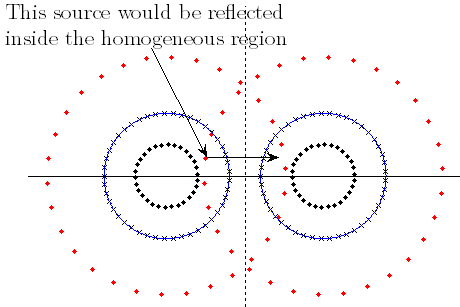Figure 4.1: A configuration in which the problem occurs if symmetry of class p=4 is used.The propagation constants should not fall exactly on the light line. A warning is issued if this happens.As mentioned previously, the SMTP cannot, at the moment, deal with sharp corners. The algorithm distributes the sources at a distance which is proportional to the minimal radius of curvature, which is zero at a corner. Corners may lead to singularities in the field and therefore may require special attention. Nevertheless, if the corner is not too sharp, reasonably good results can be obtained.

## Bibliography


A. Hochman and Y. Leviatan, "Efficient and spurious-free integral-equation-based optical waveguide mode solver," Opt. Express, pp. 14431-14453, 2007.

Z. Altman, H. Cory, and Y. Leviatan, "Cutoff frequencies of dielectric waveguides using the multifilament current model," Microw. Opt. Technol. Lett., vol. 3, no. 8, pp. 294-295, Aug. 1990.

A. Hochman and Y. Leviatan, "Analysis of strictly bound modes in photonic crystal fibers by use of a source-model technique," J. Opt. Soc. Am. A, vol. 21, no. 6, pp. 1073-1081, June 2004.

--, "Calculation of confinement losses in photonic crystal fibers by use of a source-model technique," J. Opt. Soc. Am. B, vol. 22, no. 2, Feb. 2005.

T. P. White, B. T. Kuhlmey, R. C. McPhedran, D. Maystre, G. Renversez, C. M. de Sterke, and L. C. Botten, "Multipole method for microstructured optical fibers. I. Formulation," Journal of the Optical Society of America B, vol. 19, no. 10, pp. 2322-2330, Oct. 2002.

M. Szpulak, W. Urbanczyk, E. Serebryannikov, A. Zheltikov, A. Hochman, Y. Leviatan, R. Kotynski, and K. Panajotov, "Comparison of different methods for rigorous modeling of photonic crystal fibers," Opt. Express, pp. 5699-5714, 2006.

A. Webb, F. Poletti, D. Richardson, J. Sahu, et al., "Suspended-core holey fiber for evanescent-field sensing," Optical Engineering, vol. 46, p. 010503, 2007.

P. B. Johnson and R. W. Christy, "Optical constants of the noble metals," Phys. Rev. B, vol. 6, no. 12, pp. 4370-4379, Dec 1972.

P. R. McIsaac, "Symmetry-induced modal characteristics of uniform waveguides-I: Summary of results," IEEE Transactions on Microwave Theory and Techniques, vol. 23, no. 5, pp. 421-429, May 1975.

J. M. Fini, "Improved symmetry analysis of many-moded microstructure optical fibers," Journal of the Optical Society of America B, vol. 21, no. 8, pp. 1431-1436, Aug. 2004.File translated from TEX by TTH, version 3.77.
On 13 Aug 2007, 16:47.Home | What's New | Download | User's Guide | Bibliography | License Copyright © 2007 Amit Hochman and Yehuda Leviatan, Technion - Israel Institute of Technology. Last modified: 07/06/09.